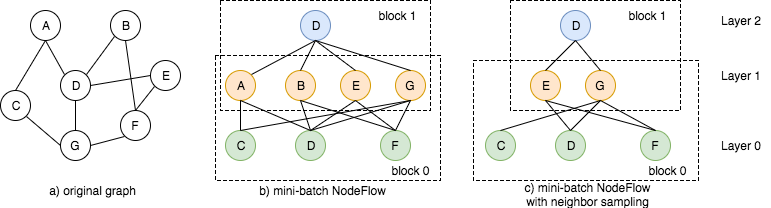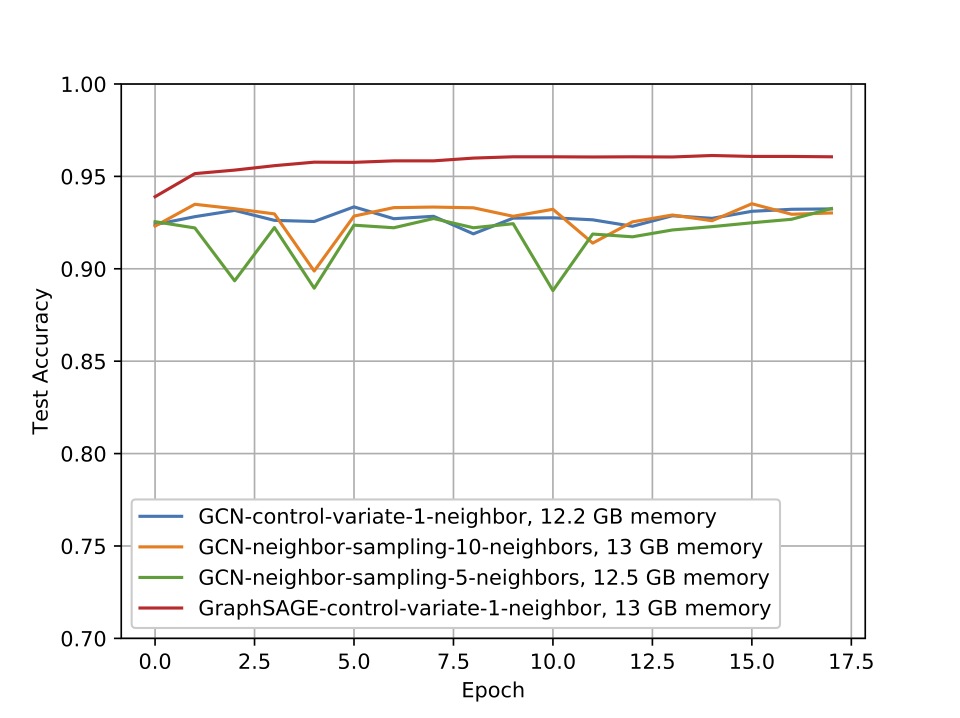# NodeFlow and Sampling¶

Author: Ziyue Huang, Da Zheng, Quan Gan, Jinjing Zhou, Zheng Zhang

Graph convolutional network ~~~

In an $$L$$-layer graph convolution network (GCN), given a graph $$G=(V, E)$$, represented as an adjacency matrix $$A$$, with node features $$H^{(0)} = X \in \mathbb{R}^{|V| \times d}$$, the hidden feature of a node $$v$$ in $$(l+1)$$-th layer $$h_v^{(l+1)}$$ depends on the features of all its neighbors in the previous layer $$h_u^{(l)}$$:

$z_v^{(l+1)} = \sum_{u \in \mathcal{N}(v)} \tilde{A}_{uv} h_u^{(l)} \qquad h_v^{(l+1)} = \sigma ( z_v^{(l+1)} W^{(l)})$

where $$\mathcal{N}(v)$$ is the neighborhood of $$v$$, $$\tilde{A}$$ could be any normalized version of $$A$$ such as $$D^{-1} A$$ in Kipf et al., $$\sigma(\cdot)$$ is an activation function, and $$W^{(l)}$$ is a trainable parameter of the $$l$$-th layer.

In the node classification task you minimize the following loss:

$\frac{1}{\vert \mathcal{V}_\mathcal{L} \vert} \sum_{v \in \mathcal{V}_\mathcal{L}} f(y_v, z_v^{(L)})$

where $$y_v$$ is the label of $$v$$, and $$f(\cdot, \cdot)$$ is a loss function, e.g., cross entropy loss.

While training GCN on the full graph, each node aggregates the hidden features of its neighbors to compute its hidden feature in the next layer.

In this tutorial, you run GCN on the Reddit dataset constructed by Hamilton et al., wherein the nodes are posts and edges are established if two nodes are commented by a same user. The task is to predict the category that a post belongs to. This graph has 233,000 nodes, 114.6 million edges and 41 categories. First load the Reddit graph.

import numpy as np
import dgl
import dgl.function as fn
from dgl import DGLGraph
from dgl.data import RedditDataset
import mxnet as mx
from mxnet import gluon

data = RedditDataset(self_loop=True)
features = mx.nd.array(data.features)
in_feats = features.shape
labels = mx.nd.array(data.labels)
n_classes = data.num_labels

# construct DGLGraph and prepare related data
g.ndata['features'] = features


Out:

Finished data loading.
NumNodes: 232965
NumEdges: 114848857
NumFeats: 602
NumClasses: 41
NumTrainingSamples: 153431
NumValidationSamples: 23831
NumTestSamples: 55703


Here you define the node UDF, which has a fully-connected layer:

class NodeUpdate(gluon.Block):
def __init__(self, in_feats, out_feats, activation=None):
super(NodeUpdate, self).__init__()
self.dense = gluon.nn.Dense(out_feats, in_units=in_feats)
self.activation = activation

def forward(self, node):
h = node.data['h']
h = self.dense(h)
if self.activation:
h = self.activation(h)
return {'activation': h}


In DGL, you implement GCN on the full graph with update_all in DGLGraph. The following code performs two-layer GCN on the Reddit graph.

# number of GCN layers
L = 2
# number of hidden units of a fully connected layer
n_hidden = 64

layers = [NodeUpdate(g.ndata['features'].shape, n_hidden, mx.nd.relu),
NodeUpdate(n_hidden, n_hidden, mx.nd.relu)]
for layer in layers:
layer.initialize()

h = g.ndata['features']
for i in range(L):
g.ndata['h'] = h
g.update_all(message_func=fn.copy_src(src='h', out='m'),
reduce_func=fn.sum(msg='m', out='h'),
apply_node_func=lambda node: {'h': layers[i](node)['activation']})
h = g.ndata.pop('h')


## NodeFlow¶

As the graph scales up to billions of nodes or edges, training on the full graph would no longer be efficient or even feasible.

Mini-batch training allows you to control the computation and memory usage within some budget. The training loss for each iteration is

$\frac{1}{\vert \tilde{\mathcal{V}}_\mathcal{L} \vert} \sum_{v \in \tilde{\mathcal{V}}_\mathcal{L}} f(y_v, z_v^{(L)})$

where $$\tilde{\mathcal{V}}_\mathcal{L}$$ is a subset sampled from the total labeled nodes $$\mathcal{V}_\mathcal{L}$$ uniformly at random.

Stemming from the labeled nodes $$\tilde{\mathcal{V}}_\mathcal{L}$$ in a mini-batch and tracing back to the input forms a computational dependency graph (a directed acyclic graph [DAG]), which captures the computation flow of $$Z^{(L)}$$.

In the example below, a mini-batch to compute the hidden features of node D in layer 2 requires hidden features of A, B, E, G in layer 1, which in turn requires hidden features of C, D, F in layer 0.For that purpose, you define NodeFlow to represent this computation flow.

NodeFlow is a type of layered graph, where nodes are organized in $$L + 1$$ sequential layers, and edges only exist between adjacent layers, forming blocks. You construct NodeFlow backwards, starting from the last layer with all the nodes whose hidden features are requested. The set of nodes the next layer depends on forms the previous layer. An edge connects a node in the previous layer to another in the next layer if the latter depends on the former. Repeat such process until all $$L + 1$$ layers are constructed. The feature of nodes in each layer, and that of edges in each block, are stored as separate tensors.

NodeFlow provides block_compute for per-block computation, which triggers computation and data propogation from the lower layer to the next upper layer.

## Neighbor sampling¶

Real-world graphs often have nodes with large degree, meaning that a moderately deep (e.g., three layers) GCN would often depend on input features of the entire graph, even if the computation only depends on outputs of a few nodes, hence its cost-ineffectiveness.

Sampling methods mitigate this computational problem by reducing the receptive field effectively. Fig-c above shows one such example.

Instead of using all the $$L$$-hop neighbors of a node $$v$$, Hamilton et al. propose neighbor sampling, which randomly samples a few neighbors $$\hat{\mathcal{N}}^{(l)}(v)$$ to estimate the aggregation $$z_v^{(l+1)}$$ of its total neighbors $$\mathcal{N}(v)$$ in $$l$$-th GCN layer, by an unbiased estimator $$\hat{z}_v^{(l+1)}$$

$\hat{z}_v^{(l+1)} = \frac{\vert \mathcal{N}(v) \vert }{\vert \hat{\mathcal{N}}^{(l)}(v) \vert} \sum_{u \in \hat{\mathcal{N}}^{(l)}(v)} \tilde{A}_{uv} \hat{h}_u^{(l)} \qquad \hat{h}_v^{(l+1)} = \sigma ( \hat{z}_v^{(l+1)} W^{(l)} )$

Let $$D^{(l)}$$ be the number of neighbors to be sampled for each node at the $$l$$-th layer, then the receptive field size of each node can be controlled under $$\prod_{i=0}^{L-1} D^{(l)}$$ by neighbor sampling.

You then implement neighbor sampling by NodeFlow:

class GCNSampling(gluon.Block):
def __init__(self,
in_feats,
n_hidden,
n_classes,
n_layers,
activation,
dropout,
**kwargs):
super(GCNSampling, self).__init__(**kwargs)
self.dropout = dropout
self.n_layers = n_layers
with self.name_scope():
self.layers = gluon.nn.Sequential()
# input layer
# hidden layers
for i in range(1, n_layers-1):
# output layer

def forward(self, nf):
nf.layers.data['activation'] = nf.layers.data['features']
for i, layer in enumerate(self.layers):
h = nf.layers[i].data.pop('activation')
if self.dropout:
h = mx.nd.Dropout(h, p=self.dropout)
nf.layers[i].data['h'] = h
# block_compute() computes the feature of layer i given layer
# i-1, with the given message, reduce, and apply functions.
# Here, you essentially aggregate the neighbor node features in
# the previous layer, and update it with the layer function.
nf.block_compute(i,
fn.copy_src(src='h', out='m'),
lambda node : {'h': node.mailbox['m'].mean(axis=1)},
layer)
h = nf.layers[-1].data.pop('activation')
return h


DGL provides NeighborSampler to construct the NodeFlow for a mini-batch according to the computation logic of neighbor sampling. NeighborSampler returns an iterator that generates a NodeFlow each time. This function has many options to give users opportunities to customize the behavior of the neighbor sampler, including the number of neighbors to sample or the number of hops to sample, for example. Please see its API document for more details.

# dropout probability
dropout = 0.2
# batch size
batch_size = 1000
# number of neighbors to sample
num_neighbors = 4
# number of epochs
num_epochs = 1

# initialize the model and cross entropy loss
model = GCNSampling(in_feats, n_hidden, n_classes, L,
mx.nd.relu, dropout, prefix='GCN')
model.initialize()
loss_fcn = gluon.loss.SoftmaxCELoss()

{'learning_rate': 0.03, 'wd': 0})

for epoch in range(num_epochs):
i = 0
for nf in dgl.contrib.sampling.NeighborSampler(g, batch_size,
num_neighbors,
neighbor_type='in',
shuffle=True,
num_hops=L,
seed_nodes=train_nid):
# When NodeFlow is generated from NeighborSampler, it only contains
# the topology structure, on which there is no data attached.
# Users need to call copy_from_parent to copy specific data,
# such as input node features, from the original graph.
nf.copy_from_parent()
# forward
pred = model(nf)
batch_nids = nf.layer_parent_nid(-1).astype('int64')
batch_labels = labels[batch_nids]
# cross entropy loss
loss = loss_fcn(pred, batch_labels)
loss = loss.sum() / len(batch_nids)
# backward
loss.backward()
# optimization
trainer.step(batch_size=1)
print("Epoch[{}]: loss {}".format(epoch, loss.asscalar()))
i += 1
# You only train the model with 32 mini-batches just for demonstration.
if i >= 32:
break


Out:

Epoch: loss 3.7190444469451904
Epoch: loss 2.898159980773926
Epoch: loss 2.429910898208618
Epoch: loss 1.9339581727981567
Epoch: loss 1.8144608736038208
Epoch: loss 1.5522640943527222
Epoch: loss 1.3704458475112915
Epoch: loss 1.404953956604004
Epoch: loss 1.2175884246826172
Epoch: loss 1.1675583124160767
Epoch: loss 1.201634407043457
Epoch: loss 1.2693356275558472
Epoch: loss 1.164099097251892
Epoch: loss 1.2306642532348633
Epoch: loss 1.068681001663208
Epoch: loss 1.0759804248809814
Epoch: loss 0.9130799770355225
Epoch: loss 0.9212628602981567
Epoch: loss 1.0682505369186401
Epoch: loss 1.0691663026809692
Epoch: loss 0.8849312663078308
Epoch: loss 0.9656017422676086
Epoch: loss 0.9721274971961975
Epoch: loss 0.9061383008956909
Epoch: loss 0.983157217502594
Epoch: loss 0.9183320999145508
Epoch: loss 0.9337270855903625
Epoch: loss 0.8921586871147156
Epoch: loss 1.0049136877059937
Epoch: loss 0.9190546870231628
Epoch: loss 0.7967264652252197
Epoch: loss 1.0008349418640137


## Control variate¶

The unbiased estimator $$\hat{Z}^{(\cdot)}$$ used in neighbor sampling might suffer from high variance, so it still requires a relatively large number of neighbors, e.g. $$D^{(0)}=25$$ and $$D^{(1)}=10$$ in Hamilton et al.. With control variate, a standard variance reduction technique widely used in Monte Carlo methods, 2 neighbors for a node seems sufficient.

Control variate method works as follows: Given a random variable $$X$$ and you wish to estimate its expectation $$\mathbb{E} [X] = \theta$$, it finds another random variable $$Y$$ which is highly correlated with $$X$$ and whose expectation $$\mathbb{E} [Y]$$ can be easily computed. The control variate estimator $$\tilde{X}$$ is

$\tilde{X} = X - Y + \mathbb{E} [Y] \qquad \mathbb{VAR} [\tilde{X}] = \mathbb{VAR} [X] + \mathbb{VAR} [Y] - 2 \cdot \mathbb{COV} [X, Y]$

If $$\mathbb{VAR} [Y] - 2\mathbb{COV} [X, Y] < 0$$, then $$\mathbb{VAR} [\tilde{X}] < \mathbb{VAR} [X]$$.

Chen et al. proposed a control variate based estimator used in GCN training, by using history $$\bar{H}^{(l)}$$ of the nodes which are not sampled, the modified estimator $$\hat{z}_v^{(l+1)}$$ is

$\begin{split}\hat{z}_v^{(l+1)} = \frac{\vert \mathcal{N}(v) \vert }{\vert \hat{\mathcal{N}}^{(l)}(v) \vert} \sum_{u \in \hat{\mathcal{N}}^{(l)}(v)} \tilde{A}_{uv} ( \hat{h}_u^{(l)} - \bar{h}_u^{(l)} ) + \sum_{u \in \mathcal{N}(v)} \tilde{A}_{uv} \bar{h}_u^{(l)} \\ \hat{h}_v^{(l+1)} = \sigma ( \hat{z}_v^{(l+1)} W^{(l)} )\end{split}$

This method can also be conceptually implemented in DGL as shown here.

have_large_memory = False
# The control-variate sampling code below needs to run on a large-memory
# machine for the Reddit graph.
if have_large_memory:
g.ndata['h_0'] = features
for i in range(L):
g.ndata['h_{}'.format(i+1)] = mx.nd.zeros((features.shape, n_hidden))
# With control-variate sampling, you only need to sample two neighbors to train GCN.
for nf in dgl.contrib.sampling.NeighborSampler(g, batch_size, expand_factor=2,
neighbor_type='in', num_hops=L,
seed_nodes=train_nid):
for i in range(nf.num_blocks):
# aggregate history on the original graph
g.pull(nf.layer_parent_nid(i+1),
fn.copy_src(src='h_{}'.format(i), out='m'),
lambda node: {'agg_h_{}'.format(i): node.mailbox['m'].mean(axis=1)})
nf.copy_from_parent()
h = nf.layers.data['features']
for i in range(nf.num_blocks):
prev_h = nf.layers[i].data['h_{}'.format(i)]
# compute delta_h, the difference of the current activation and the history
nf.layers[i].data['delta_h'] = h - prev_h
# refresh the old history
nf.layers[i].data['h_{}'.format(i)] = h.detach()
# aggregate the delta_h
nf.block_compute(i,
fn.copy_src(src='delta_h', out='m'),
lambda node: {'delta_h': node.data['m'].mean(axis=1)})
delta_h = nf.layers[i + 1].data['delta_h']
agg_h = nf.layers[i + 1].data['agg_h_{}'.format(i)]
# control variate estimator
nf.layers[i + 1].data['h'] = delta_h + agg_h
nf.apply_layer(i + 1, lambda node : {'h' : layer(node.data['h'])})
h = nf.layers[i + 1].data['h']
# update history
nf.copy_to_parent()


You can see full example here, MXNet code and PyTorch code.

Below shows the performance of graph convolution network and GraphSage with neighbor sampling and control variate sampling on the Reddit dataset. Our GraphSage with control variate sampling, when sampling one neighbor, can achieve over 96 percent test accuracy.## More APIs¶

In fact, block_compute is one of the APIs that comes with NodeFlow, which provides flexibility to research new ideas. The computation flow underlying a DAG can be executed in one sweep, by calling prop_flows.

prop_flows accepts a list of UDFs. The code below defines node update UDFs for each layer and computes a simplified version of GCN with neighbor sampling.

apply_node_funcs = [
lambda node : {'h' : layers(node)['activation']},
lambda node : {'h' : layers(node)['activation']},
]
for nf in dgl.contrib.sampling.NeighborSampler(g, batch_size, num_neighbors,
neighbor_type='in', num_hops=L,
seed_nodes=train_nid):
nf.copy_from_parent()
nf.layers.data['h'] = nf.layers.data['features']
nf.prop_flow(fn.copy_src(src='h', out='m'),
fn.sum(msg='m', out='h'), apply_node_funcs)


Internally, prop_flow triggers the computation by fusing together all the block computations, from the input to the top. The main advantages of this API are 1) simplicity, 2) allowing more system-level optimization in the future.

Total running time of the script: ( 4 minutes 55.190 seconds)

Gallery generated by Sphinx-Gallery